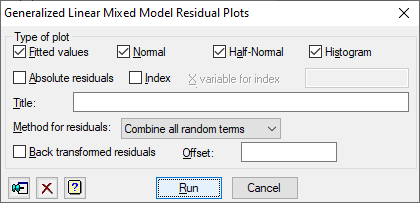1. Home
2. Generalized Linear Mixed Model Residual Plots

# Generalized Linear Mixed Model Residual Plots

The dialog plots the residuals from a generalized linear mixed model analysis.## Type of plot

The check boxes in this dialog allow you to select up to 4 types of plot of residuals from a generalized linear mixed model analysis:

 Fitted values Residuals versus fitted values Normal Normal plot Half-Normal Half-Normal plot Histogram Histogram of residuals Absolute residuals The absolute values of the residuals versus the fitted values Index The residuals versus the given x variate

## Title

This provides a title for the plot. If this is not provided, a title will be automatically generated.

## Method for residuals

The list allows selection from type of residuals that can be used.

 Combine all random terms Use the residuals combined from all random terms. Final random term only Use the residuals from the final random term.

## Back transformed residuals

If this is ticked, residuals on the natural scale (calculated using back-transformed fitted values) will be used in the plots, otherwise standardized residuals on the linear-predictor scale will be used.

## Offset

Value of offset to use when calculating the residuals; if this is not set a default value of 0 will be used.